### Home > CALC > Chapter 3 > Lesson 3.3.4 > Problem3-134

3-134.
1. For each of the following, find the second derivative with respect to x. Homework Help ✎

1. y = 8x99

2.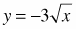3.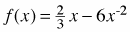4. f(x) = 7 − 2 cos x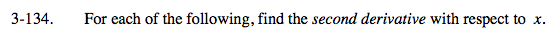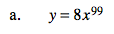$y=8x^{99}$

$\frac{dy}{dx}=(99)8x^{98}=792x^{98}$

$\frac{d^{2}y}{dx^{2}}=\underline{ \ \ \ \ \ \ \ }$

(98)(99)8x97 = 77,616x97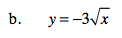Refer to part (a) for further guidance. Remember to rewrite the square root with an exponent before proceeding.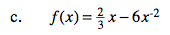Refer to part (a) for further guidance.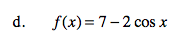Refer to part (a) for further guidance.